### 2015 SSC CGL Tier 2 Quantitative Aptitude 12 Apr Question Paper with Answer Key

1. If the product of two positive numbers be 1575 and their ratio is 7:9, then the greater number is
(A) 35
(B) 63
(C) 45
(D) 135

2. The average weight of first 11 persons among 12 persons is 95 kg. The weight of 12th person is 33 kg more than the average weight of all the 12 persons. The weight of the 12th person is
(A) 128 kg
(B) 97.45 kg
(C) 128.75 kg
(D) 131 kg

3. The average of the largest and smallest 3 digit numbers formed by 0, 2 and 4 would be
(A) 213
(B) 303
(C) 312
(D) 222

4. The average of six numbers is 3.95. The average of two of them is 3.4, while the average of the other two is 3.85. The average of the remaining two numbers is
(A) 4.5
(B) 4.7
(C) 4.6
(D) 4.8

5. If the average of eight consecutive even numbers be 93, then the greatest number among them is
(A) 86
(B) 98
(C) 100
(D) 102

6. If the arithmetic mean of 3a and 4b is greater than 50, and a is twice b, then the smallest possible integer value of a is
(A) 18
(B) 19
(C) 20
(D) 21

7. The average (arithmetic mean) of 330,360 and 390 is
(A) 360
(B) 3177
(C) 327 + 357 + 387
(D) 329 + 359 + 389

8. Pooja wants to sell a watch at a profit of 20%. She bought it at 10% less and sold it at Rs. 30 less, but still she gained 20%. The cost price of watch is
(A) Rs. 220
(B) Rs. 225
(C) Rs. 240
(D) Rs. 250

9. A shopkeeper sold his goods at half the list price and thus lost 20%. If he had sold on the listed price, his gain percentage would be
(A) 20%
(B) 35%
(C) 60%
(D) 72%

10. Two types of tea costing Rs. 180/kg and Rs. 280/kg should be mixed in the ratio so that the mixture obtained, sold at Rs. 320/kg to earn a profit of 20% is
(A) 1:13
(B) 2:13
(C) 3:13
(D) 4:13

11. A profit of 12% is made when ‘a mobile phone is sold at Rs. P and there is 4% loss when the phone is sold at Rs. Q. Then Q: P is
(A) 4:5
(B) 3:1
(C) 1:1
(D) 6:7

12. A sells a cycle to B at a profit of 20% and B sells it to C at a loss of 25%. If C bought the cycle for Rs. P, then the cost price of it for A was
(A) Rs. 9/10 P
(B) Rs. 10/9 P
(C) Rs. 1/20 P
(D) Rs. 9/20 P

13. A man buys a toy for Rs. 25 and sells it for Rs. 30. His gain percent is
(A) 5%
(B) 2.5%
(C) 20%
(D) 10%

14. From 1980-1990, the population of a country was increased by 20%.
From 1990-2000, the population of the country was increased by 20%.
From 2000-2010 the population of the country was increased by 20%. Then the overall increased population (in percentage) of the country from 1980-2010 was
(A) 60%
(B) 62.8%
(C) 72.2%
(D) 72.8%

15. A supply of juice lasts for 35 days. If its use is increased by 40% the number of days would the same amount of juice lasts, is
(A) 30 days
(B) 27 days
(C) 25 days
(D) 24 days

16. A store offers a variety of discounts that range between 20% and 25% inclusive. If a book is discounted to a price of Rs. 270, then its greatest possible original price was
(A) Rs.324
(B) Rs.337.5
(C) Rs.345.5
(D) Rs.360

17. If the ratio of cost price to selling price is 10 : 11, then the rate of percent of profit is
(A) 0.1%
(B) 1%
(C) 1.1%
(D) 10%

18. A boat takes half time in moving a certain distance downstream than upstream. The ratio of the speed of the boat in still water and that of the current is
(A) 1:2
(B) 3:1
(C) 2:1
(D) 4:3

19. A tank has two pipes. The first pipe can fill it in 4 hours and the second can empty it in 16 hours. If two pipes be opened together at a time, then the tank will be filled in
(A) 10 hours
(B) 5 1/3 hours
(C) 5 1/2 hours
(D) 6 hours

20. A farmer travelled a distance of 61 km in 9 hours. He travelled partly on foot at the rate 4 km/hour and partly on bicycle at the rate 9 km/hour. The distance travelled on foot is
(A) 14 km
(B) 15 km
(C) 16 km
(D) 17 km

21. A man rows upstream 36 km and downstream 48 km taking 6 hours each time. The speed of the current is
(A) 2 km/hour
(B) 1.5 km/hour
(C) 0.5 km/hour
(D) 1 km/hour

22. On a certain principal the compound interest compounded annually for the second year at 10% per annum is Rs. 132. The principal is
(A) Rs.1000
(B) Rs.1320
(C) Rs.1250
(D) Rs.1200

23. The principal which gives Rs. 1 interest per day at a rate of 5% simple interest per annum is
(A) Rs. 7300
(B) Rs. 3650
(C) Rs. 5000
(D) Rs. 36500

24. When principal = Rs. S, rate of interest = 2r % p. a, then a person will get after 3 years at compound interest
(A) Rs. S(1+r/100)3
(B) Rs. 3S(1/r/100)3
(C) Rs. 6Sr/100
(D) Rs. S(1+r/50)3

25. The sum of money which becomes Rs.2420 at 10% rate of compound interest after two years is
(A) Rs.1000
(B) Rs.1500
(C) Rs.2000
(D) Rs.2500

26. From a solid right circular cylinder of length 4 cm and diameter 6 cm, a conical cavity of the same height and base is hollowed out. The whole surface of the remaining solid (in square cm.) is
(A) 15π
(B) 24 π
(C) 48 π
(D) 63 π

27. A spherical ball of radius 1 cm is dropped into a conical vessel of radius 3 cm and slant height 6 cm. The volume of water (in cm3), that can just immerse the ball, is
(A) π /3
(B) 4 π/3
(C) 5 π/3
(D) 3 π

28. The in-radius of a triangle is 6 cm, and the sum of the lengths of its sides is 50 cm. The area of the triangle (in sq. cm.) is
(A) 50
(B) 56
(C) 150
(D) 300

29. One of the angles of a right-angled triangle is 15°, and the hypotenuse is 1m. The area of the triangle (in sq. cm.) is
(A) 1200
(B) 1215
(C) 1220
(D) 1250

30. Assume that a drop of water is spherical and its diameter is one- tenth of a cm. A conical glass has a height equal to the diameter of its rim. If 32000 drops of water fill the grass completely, ‘then the height of the glass (in cm.) is
(A) 1
(B) 2
(C) 3
(D) 4

31. If the height of a cylinder is 4 times its circumference, the volume of the cylinder in terms of its circumference c, is
(A) 4πc3
(B) 2πc3
(C) 2c3/π
(D) c3/π

32. If the ratio of the angles of a quadrilateral is 2:7:2:7, then it is a
(A) parallelogram
(B) rhombus
(C) trapezium
(D) square

33. If for an isosceles triangle the length of each equal side is ‘a’ units and that of the third side is ‘b’ units, then its area will be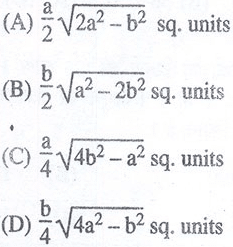34. The outer and inner diameter of a circular path be 728 m and 700 m respectively. The breadth of the path is
(A) 28 m
(B) 20 m
(C) 7 m
(D) 14 m

35. A piece of wire when bent to form a circle will have a radius of 84 cm. If the wire is bent to form a square, the length of a side of the square is
(A) 132 cm
(B) 225 cm
(C) 152 cm
(D) 168 cm

36. If radius of a circle is increased by 5%, then the increase in it’s area is
(A) 10%
(B) 5%
(C) 10.25%
(D) 5.75%

37. The area of the parallelogram whose length is 30 cm, width is 20 cm and one diagonal is 40 cm is
(A) 100√15 cm2
(B) 150√15 cm2
(C) 200√15 cm2
(D) 300√15 cm2

38. On increasing each side of a square by 50%, the ratio of the area of new formed square and the given square will be
(A) 9:3.5
(B) 9:4
(C) 9:5
(D) 9:7

39. The area of a circle is 324π sq.cm. The length of its longest chord (in cm.) is
(A) 28
(B) 32
(C) 36
(D) 38

40. The area of a rhombus is 256.sq.cm. and one of its diagonal is twice the other in length. Then length of its larger diagonal is
(A) 16 cm
(B) 24 cm
(C) 32 cm
(D) 48 cm

41.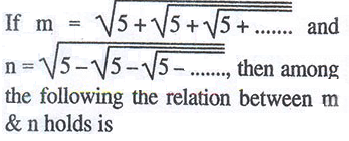(A) m + n – 1 = 0
(B) m – n – 1 = 0
(C) m – n + 1 = 0
(D) m + n + 1 = 0

42. If 3-5x/2x+3-5y/2y+3-5z/2z= 0, then the value of 2/x+2/y+2/z is
(A) 5
(B) 15
(C) 20
(D) 10

43. The distance between 2 places R and S is 42 km. Anita starts from R with a uniform speed of 4km/h towards S and at the same time Romita starts from S towards R also with same uniform speed. They meet each other after 6 hours. The speed of Romita is
(A) 6 km/hour
(B) 8 km/hour
(C) 18 km/hour
(D) 20 km/hour

44. If the side of a square is 1/2 (x + 1) units and its diagonal is 3-x/√2 units, then the length of the side of the square would be –
(A) 1/2 units
(B) 2 units
(C) 4/3 units
(D) 1 units

45. If 2s = a + b + c, then the value of s(s – c) + (s – a) (s – b) is
(A) abc
(B) a + b + c/2
(C) ab
(D) 0

46. If p + m = 6 and p3 + m3 = 72, then the value of pm is
(A) 12
(B) 8
(C) 6
(D) 9

47. When xm is multiplied by xn, product is 1. The relation between m and n is
(A) m = n
(B) m = -n
(C) mn = 1
(D) m + n = 1

48. The area (in sq. unit) of the triangle formed by the graphs of the equations x = 4, y = 3 and 3.r + 4y = 12; is
(A) 12
(B) 3
(C) 24
(D) 6

49. If 2p/pr-2p + 1 = ¼, then the value of (P+1/p)is
(A) 2/5
(B) 10
(C) 7
(D) 1

50. If the ordinate and abscissa of the point (Kr, 2K – 1) be equal, then the value of K is
(A) -1
(B) ½
(C) 0
(D) 1

51. ABC is a triangle in which DE || BC and AD:DB = 5:4 . Then DE: BC is
(A) 4:9
(B) 5:9
(C) 4:5
(D) 9:5

52. The radii of two concentric circles are 17 cm and 25 cm. A straight line PQRS intersects the larger circle at the points P and S and intersects the smaller circle at the points Q and R. If QR = 16 cm, then the length (in cm.) of PS is
(A) 32
(B) 40
(C) 41
(D) 33

53. AB is a diameter of a circle with, centre 0. The tangents at C meets AB produced at Q. If ∠CAB = 34°, then measure of ∠CBA is
(A) 34°
(B) 124°
(C) 56°
(D) 68°

54. For art equilateral triangle, the ratio of the in-radius and the outer-radius is
(A) 1:√2
(B) 1:√3
(C) 1:2
(D) 1:3

55. If a and b are the lengths of the sides of a right triangle whose hypotenuse is 10 and whose area is 20, then the value of (a + b)2 is
(A) 180
(B) 160
(C) 140
(D) 120

56. The circumference of a triangle is 24 cm and the circumference of its in-circle is 44 cm. Then the area of the triangle is (taking π = 22/7)
(A) 84 square cm.
(B) 68 square cm.
(C) 56 square cm.
(D) 48 square cm.

57. If the length of each of two equal sides of an isosceles triangle is 10 cm. and the adjacent angle is 45°, then the area of the triangle is
(A) 12√2 square cm.
(B) 15√2 square cm.
(C) 20√2 square cm.
(D) 25√2 square cm.

58. Let P and Q be two points on a circle with centre O. If two tangents of the circle through P and Q meet at A with ∠PAQ = 48°, then ∠APQ is
(A) 48°
(B) 60°
(C) 96°
(D) 66°

59. The length of the diagonal of a rectangle with sides 4 m and 3 m would be
(A) 7 m
(B) 14 m
(C) 12 m
(D) 5 m

60. If the sides of a triangle are in the ratio 3 : 1 ¼ : 3 1/4, then the triangle is
(A) Obtuse triangle
(B) Acute triangle
(C) Right triangle
(D) Equiangular triangle

61. The value of Ө (0 ≤ Ө ≤ 90°) satisfying 2 sin2 Ө = 3 cos Ө is
(A) 30°
(B) 45°
(C) 60°
(D) 90°

62. a, b, c are the lengths of three sides of a triangle ABC. If a, b, c are related by the relation a2 + b2 + c2 = ab + be + ca, then the value of (sin2A + sin2B + sin2C) is
(A) 3√3/2
(B) 9/4
(C) ¾
(D) 3/2

63. If a sin Ө + b cos Ө = c, then a cos Ө – b sin Ө is equal to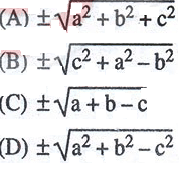64. If sin Ө + cos Ө = √2 sin (90° – Ө) then the value of cot Ө is
(A) √2-1
(B) -√2+1
(C) -√2- 1
(D) √2+1

65. If Ө is positive acute angle and 3(sec2Ө + tan2Ө) = 5, then the value of cos 2Ө is
(A) 1/√2
(B) 1
(C) ½
(D) √3/2

66. If x cos230° .sin 60° = tan245° – sec 60°/ cosec 60° then the value of x is
(A) 1/√2
(B) ½
(C) 1/√3
(D) 2 2/3

67. If tan a = 2, then the value of cosec2a – sec2a/ cosec2a + sec2a is
(A) -3/5
(B) 17/5
(C) -15/9
(D) 3/5

68. If sin (Ө + 30°) = 3/√12 , then the value of cos2Ө is
(A) √3/2
(B) ½
(C) ¼
(D) ¾

69. In a right angled triangle ΔPQR, PR is the hypotenuse of length 20 cm, ∠PRQ = 30°, the area of the triangle is
(A) 100√3 cm2
(B) 100/√3cm2
(C) 50√3 cm2
(D) 25√3 cm2

70. If 0≤Ө≤ 90° and 4 cos2Ө-4√3 cos Ө + 3 = 0, then the value of Ө is
(A) 45°
(B) 60°
(C) 30°
(D) 90°

71. If x: y = 3 :2, then value of x+y/x-y is
(A) 1:3
(B) 3:1
(C) 5:1
(D) 1:5

72. If 50% of x = 30% y, then x: y is
(A) 5 : 3
(B) 3 :5
(C) 2: 3
(D) 3 : 2

73.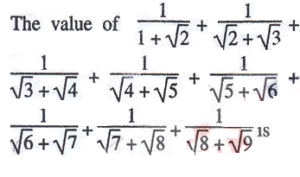(A) 0
(B) 1
(C) 2
(D) 4

74. While solving a problem, by mistake, Anita squared a number and then subtracted 25 from it rather than first subtracting 25 from the number and then squaring it. But she got the right answer. What was the given number?
(A) 13
(B) 38
(C) 48
(D) Cannot be determined

75. A General of Army wants to form a square from 36562 armies. After arrangement, he found some armies left. How many armies were left?
(A) 36
(B) 65
(C) 81
(D) 97

76. Square root of 2+√3/2 is
(A) ± 1/2(√3-2)
(B) ± 1/2(√3-1)
(C) ± 1/√2(√3+1)
(D) None of these

77. the value of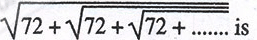(A) 8
(B) 12
(C) 9
(D) 18

78. In a farm there are cows and hens. If heads are counted there are 180, if legs are counted there are 420. The number of cows in the farm is
(A) 150
(B) 30
(C) 130
(D) 50

79. The number which can be written in the form of n (n + 1) (n + 2), where n is a natural number, is
(A) 3
(B) 6
(C) 7
(D) 5

80. The value of (11111)2 is
(A) 121212121
(B) 11344311
(C) 12344321
(D) 123454321

81. Two workers A and B are engaged to do a piece of work. A working alone would take 8 hours more to complete the work than when work together. If B worked alone, would take 4 -1/2 hours more than when work together. The time required to finish the work together is
(A) 4 hours
(B) 6 hours
(C) 5 hours
(D) 8 hours

82. If a man earns Rs. 2000 for his first 50 hours of work in a week and is then paid one and one half times his regular hourly rate for any additional hours, then the hours must he work to make Rs.2300 in a week is
(A) 4 hours
(B) 5 hours
(C) 6 hours
(D) 7 hours

83. If x can finish a job in 4 hours and y can finish the same job in 8 hours independently, then they together will finish the job in
(A) 160 minutes
(B) 150 minutes
(C) 140 minutes
(D) 120 minutes

84. A company employed 200 workers to complete a certain work in 150 days.
If only 1/4th of the work has been done in 50 4ays, then in order to complete the whole work in time, the number of additional workers to be employed was
(A) 300
(B) 200
(C) 100
(D) 600

85. x can copy 80 pages in 20 hours, x and y together can copy 135 pages in 27 hours. Then y can copy 20 pages in
(A) 3 hours
(B) 12 hours
(C) 20 hours
(D) 24 hours

86. x does 1/4 of a job in 6 days, y completes rest of the job in 12 days. Then x and y could complete the job together in
(A) 9 3/5 days
(B) 7 1/3 days
(C) 9 days
(D) 8 1/8 days

87. Mr. x and Mr. y each bought the same motorcycle using a 10% off coupon. Mr. x’s cashier took ,10% off the price and then added 8.5% sales tax whereas Mr. y’s cashier first added the sales tax and then took 10% off the total price. The amount Mr. x paid is
(A) same as the amount Mr. y paid
(B) greater than Rs. 850 as the amount Mr. y paid
(C) less than Rs. 550 as the amount Mr. y paid
(D) greater than Rs. 85 as the amount Mr. y paid

88. If a person marked a product 25% above the cost price but allows 10% discount, then the percentage of^ profit is
(A) 15%
(B) 12.5%
(C) 35%
(D) 17.5%

89. The price of an antique is reduced by 20% and then this price is again reduced by 10%. The total reduction of the price is
(A) 30%
(B) 28%
(C) 25%
(D) 23%

90. A builder purchases 25 windows at 25% off the total price of Rs. 1,20,000. If the builder receives an additional discount of Rs. 7500 for the purchase, then the cost of each window is
(A) Rs. 3100
(B) Rs.3200
(C) Rs. 3300
(D) Rs.3400

91. If A : B = ½:1/3, B:C = 1/5 :1/3, then (A +B): (B + C) is equal to
(A) 9:10
(B) 6:15
(C) 5:8
(D) 15:16

92. In a partnership business, B’s capital was half of A’s. If after 8 months, B withdrew half of his capital and after 2 months more A withdrew 1/4th of his capital, then the. profit ratio pf A and B will be
(A) 10:23
(B) 23:10
(C) 5:2
(D) 2:5

93. A school group charters three identical buses and occupies 4/5 of the seats. After ¼ of passengers leave, the remaining passengers use only two of the buses. The fraction of the seats on the two buses that are now occupied is
(A) 7/10
(B) 9/10
(C) 8/9
(D) 7/9

94. A and B invest in the ratio 3:5. After 6 months, C joins the business , investing an amount equal to B’s. At the end of the year what will be the ratio of their profits ?
(A) 3:5:2
(B) 3:5:5
(C) 6:10:5
(D) 8:10:5

95. In a library the ratio of story books and other books is 7 : 2 and there are 1512 story books. Due to collection of some more story books the said ratio becomes 15:4. The number of story books collected is
(A) 100
(B) 97
(C) 108
(D) 205

Study the bar diagram and answer Question Nos. 96 & 97 :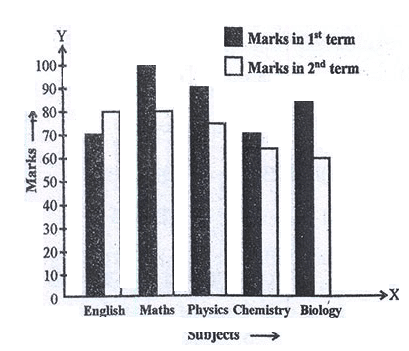96. Ratio of highest and lowest marks obtained in first term among all the subjects is
(A) 7:9
(B) 9:7
(C) 10:7
(D) 7:10

Study the bar diagram and answer Question Nos. 96 & 97 :97. Average marks obtained by the students for all subjects in second term is
(A) 65
(B) 73
(C) 62
(D) 72

In an Institution there are 800 students. Students use different modes of transport for going to the institution and return. The given pie diagram represents the requisite data. Study the diagram carefully and answer the question nos. 98 to 100.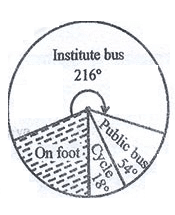98. Number of students travel in public bus is
(A) 150
(B) 120
(C) 130
(D) 125

In an Institution there are 800 students. Students use different modes of transport for going to the institution and return. The given pie diagram represents the requisite data. Study the diagram carefully and answer the question nos. 98 to 100.99. Number of students who do not use institute bus is
(A) 330
(B) 350
(C) 480
(D) 320

In an Institution there are 800 students. Students use different modes of transport for going to the institution and return. The given pie diagram represents the requisite data. Study the diagram carefully and answer the question nos. 98 to 100.100. Number of ‘students who go to institute on foot is —
(A) 160
(B) 170
(C) 120
(D) 106